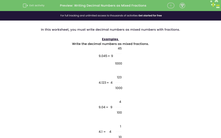# Write Decimal Numbers as Mixed Fractions

In this worksheet, students will write decimal numbers as mixed fractions.Key stage:  KS 3

Curriculum topic:   Number

Curriculum subtopic:   Work Interchangeably with Terminating Decimals and Fractions

Popular topics:   Decimals worksheets

Difficulty level:#### Worksheet Overview

In this activity, we will write decimal numbers as mixed numbers with fractions.

Remember that the numbers on the left-hand side of the decimal point are whole numbers, and the numbers on the right-hand side are tiny parts of a number.

We also need to remember that the numbers on the right-hand side of the decimal point go in the order tenths, hundredths, thousandths etc.

Examples

Write the decimal numbers as mixed fractions.

9.045 = 9 whole numbers and 045 thousandths.

9.045 = 9 45/1000

4.123 = 4 whole numbers and 123 thousandths.

4.123 = 4 123/1000

9.04 = 9 whole numbers and 04 hundredths.

9.04 = 9 4/100

4.1 = 4 whole numbers and 1 tenth.

4.1 = 4 1/10

This might look a  bit scary, but it's actually not that tricky if you look carefully at the place value of the numbers on the right-hand side of the decimal point.

We've included a place value chart here in case it's helpful!Over to you now with the questions!

### What is EdPlace?

We're your National Curriculum aligned online education content provider helping each child succeed in English, maths and science from year 1 to GCSE. With an EdPlace account you’ll be able to track and measure progress, helping each child achieve their best. We build confidence and attainment by personalising each child’s learning at a level that suits them.

Get started